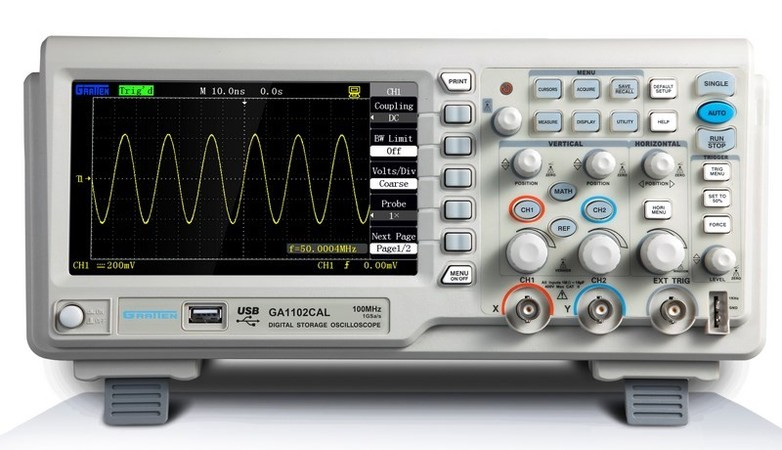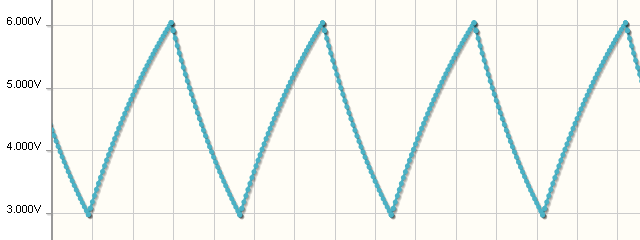top of page
•UFRJ Nautilus

# How to make a simple function generator with an Arduino

Updated: May 7, 2021

A function generator (or signal generator) is a device that generates electrical signals with different shapes, frequencies, and amplitudes. Among these shapes, square waves, triangular waves, sawtoothed waves, and sinusoidal waves are the most common that can vary the frequencies and peak-to-peak voltage. Learn here how to generate the main waves in a practical way using an Arduino.

A workbench function generator is really useful for many applications, mostly to simulate and check an electronic circuit. Its main use occurs in oscillating circuits, filters, and amplifiers.Function generator

We use an oscilloscope to make usage signals that are being received over time. With an oscilloscope, we can look at the shape of a wave at a point of the circuit.Oscilloscope with sensorial waves

There is a problem in using a signal generator: a quality device costs from \$180, in other words, it has a high cost when its need for use is punctual and simple. In that case, it's possible to an Arduino works as a basic generator of functions and accessible through your PWM outputs. It allows us to make tests for simple circuits in a fast, cheap, and practical way.

See below the design of the main waveforms.

### 1. Square Wave:The square wave is a digital signal that works with alternating logic levels: 1(maximum amplitude) and 0 (minimum amplitude). It is used as a time reference in clock signals and computing in the serial transmission of information on computer networks.

Reproducing this type of wave in Arduino is very simple, there is the tone() function that is responsible for the generation of square waves, just needing to make clear the output pin and the desired frequency of the signal, as can be seen in the example below with a frequency 50 Hz.```// Project 20 - Arduino as a frequency generator (square wave)

void setup() {
Serial.begin(9600); // Starts the Serial Monitor
}

void loop() {
// Prints the generated frequency on the screen
Serial.println("the frequency is: 50 Hz");
{
tone(6, 50); //define the pin in OUTPUT, and the frequency generated in the pin
delay (2000); //Generates the frequency for 2 seconds
}
}```

### 2. Triangular Wave:The triangular wave has a linear descent to its maximum amplitude and a linear decay to its minimum amplitude. It is normally used in sound synthesizers.

The problem with generating this waveform is that it can easily make noise. So it always needs to place some type of filter between the signal produced and the circuit in which it will be used. With the Arduino, an RC circuit solves this problem.```// Triangular Wave

int outputPWM;
int x;

#define outputPWM 3

void setup()
{
pinMode(outputPWM, OUTPUT)
}

void loop()
{
for (x=0; x<255; x++)
{
analogWrite (outputPWM, x);
delay(10);
}
for (x=255; x>0; x--)
{
analogWrite (outputPWM, x);
delay(10);
}
}
//This code is followed by an RC circuit at the PWM output of the arduino```

### 3. Sawtooth Wave:The sawtooth wave is a particularity of the triangular wave, where the rise time or the descent time is equal to zero, acting right at that moment as a "digital signal".

The applications and the code are the same only with the change of the rise or fall delay() closer to zero.```// Sawtooth Wave

int outputPWM;
int x;

#define outputPWM 3

void setup()  {
pinMode(outputPWM, OUTPUT);
}

void loop ()  {
for (x=0; x<255; x++) {
analogWrite (outputPWM, x);
delay(10);
}
for (x=255; x>0; x--) {
analogWrite (outputPWM, x);
delay(1);
}
}```

### 4. Sinusoidal Wave:The sinusoidal wave is generated from a sine or cosine function, reversing the polarity according to the frequency, for this reason, it's known as alternating current. It has several applications. In electronics, the main one is as carrier wave in radio modulations.

As in the triangular wave, noise can be found, so an RC filter is required before using it. The Arduino code is similar to the triangular one, but it has an addition of the sin() function for data collection.```// Sine wave generator simulator

int outputPWM;
int x, valor;
float seno;

#define outputPWM 3

void setup() {
pinMode (outputPWM, OUTPUT);

}

void loop () {
for (x=0; x<255; x++) {
seno = 100+(100*(sin(x*(6.28/255))));
valor = int(seno);
analogWrite(outputPWM, valor);
delay(10);
}
}```

Written by Lucas Alexandre